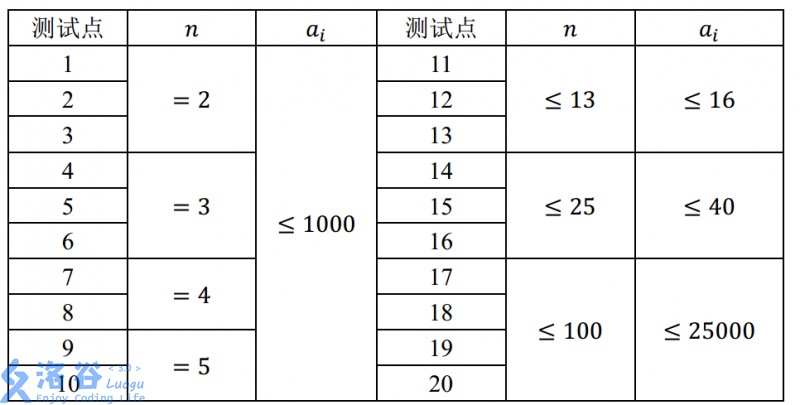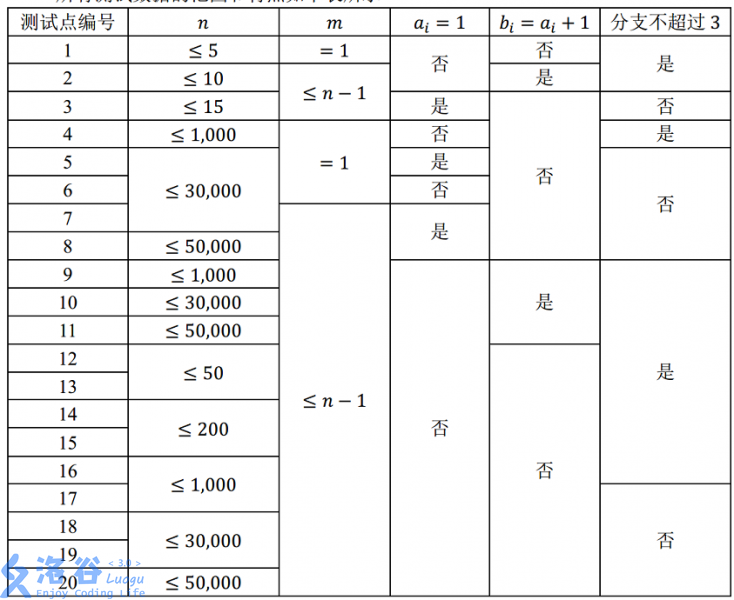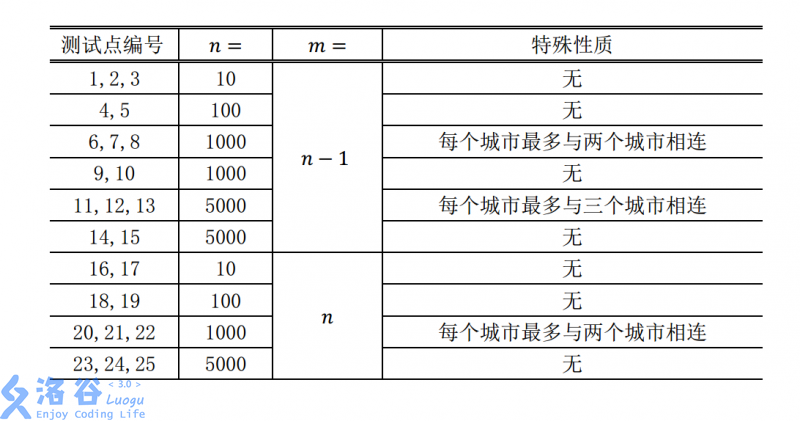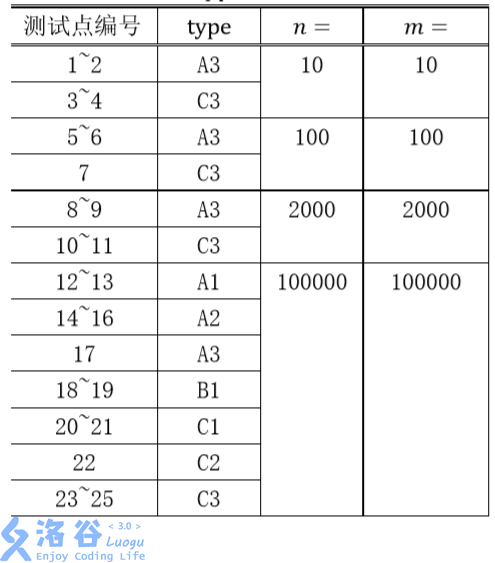day0day1

数据范围

• 对于30%的数据，$n\le 10$
• 对于70%的数据，$n\le 1000$
• 对于100%的数据，$n\le 100,000$

思路货币系统(money)

题意

$n$种货币，每种面额已知且都有无限张，求与其等价的货币系统的货币种数的最小值。等价指一个数要么两个系统都能表示，要么都不能表示。

数据范围赛道修建(track)

数据范围思路

11点多发现菊花树写错了，要改成multiset贪心。

day2

旅行(travel)

数据范围思路

$\mathcal O(n)$贪心确实是可做的，然而并没有必要。有些人在dfs里面sort，$\mathcal O(n^2\log n)$，完美卡常。

填数游戏(game)

题意

$n\times m$的矩阵填上01，对于一个从左上走到右下的方案$P$，用D/R表示走的方向，可以得到一个走法字符串$w(P)$和一个数字字符串$s(P)$。一个矩阵合法，仅当任意两条路径$P_1,P_2$，如果$w(P_1)>w(P_2)$，那么必须满足$s(P_1)\le s(P_2)$。求合法的矩阵数。

数据范围

1~4 3 3
5~10 2 1,000,000
11~13 3 1,000,000
14~16 8 8
17~20 8 1,000,000

保卫王国(defense)

数据范围• A：树为一条链。
• B：树深不超过100。
• C：树形态无特殊约束。
• 1：询问时保证要求选择1号点。
• 2：询问时保证两个点相邻。
• 3：询问无特殊约束。

思路

$\mathcal O(n^2)$暴力随便树形DP一下。想了一会儿，并没想到链的做法。

几天之后road money track travel game defense 总分
100 80 55 100 50 44 429